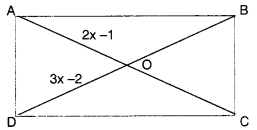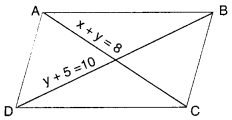Check the below Online Education NCERT MCQ Questions for Class 8 Maths Chapter 3 Understanding Quadrilaterals with Answers Pdf free download. MCQ Questions for Class 8 Maths with Answers were prepared based on the latest exam pattern. We have provided Understanding Quadrilaterals Class 8 Maths MCQs Questions with Answers to help students understand the concept very well. https://ncertmcq.com/mcq-questions-for-class-8-maths-with-answers/

Students can also refer to NCERT Solutions for Class 8 Maths Chapter 3 Understanding Quadrilaterals for better exam preparation and score more marks.

## Online Education for Understanding Quadrilaterals Class 8 MCQs Questions with Answers

Understanding Quadrilaterals Class 8 MCQ Question 1.
What is the number of sides of a triangle ?
(a) 1
(b) 2
(c) 3
(d) 4.

Class 8 Maths Chapter 3 MCQ Question 2.
What is the number of vertices of a triangle ?
(a) 1
(b) 2
(c) 3
(d) 4.

Maths Class 8 Chapter 3 MCQ Question 3.
What is the number of sides of a quadrilateral ?
(a) 1
(b) 2
(c) 3
(d) 4.

Class 8 Maths Ch 3 MCQ Question 4.
What is the number of vertices of a quadrilateral ?
la) 1
(b) 2
(c) 3
(d) 4.

MCQ On Understanding Quadrilaterals Class 8 Question 5.
How many diagonals does a quadrilateral have ?
(a) 1
(b) 2
(c) 3
(d) 4.

MCQ Questions For Class 8 Maths Chapter 3 Question 6.
The sum of the measures of all the three angles of a triangle is
la) 90°
(b) 180°
(c) 360°
(d) 720°.

Class 8 Math Chapter 3 MCQ Question 7.
The sum of the measures of all the four angles of a quadrilateral is
(a) 90°
(b) 180°
(c) 360°
(d) 720°.

MCQ On Quadrilaterals Class 8 Question 8.
How many diagonals does a triangle have ?
(a) 0
(b) 1
(c) 2
(d) 4.

Understanding Quadrilaterals MCQ Class 8 Question 9.
How many diagonals does a regular hexagon have ?
(a) 2
(b) 0
(c) 4
(d) 9

MCQ Class 8 Maths Chapter 3 Question 10.
The angle sum of a convex polygon with number of sides 7 is
(a) 900°
(b) 1080°
(c) 1440°
(d) 720°.

Hint:
n = 7
(n – 2) 180° = 900°.

Ncert Class 8 Maths Chapter 3 MCQ Questions Question 11.
The angle sum of a convex polygon with number of sides 8 is
(a) 720°
(b) 900°
(c) 1080°
(d) 1440°.

Hint:
n = 8
(n – 2) 180° = 1080°.

Class 8 Chapter 3 Maths MCQ Question 12.
The angle sum of a convex polygon with number of sides 10 is
(a ) 720°
(b) 900°
(c) 1080°
(d) 1440°.

Hint:
n = 10
(n – 2) 180° = 1440°.

MCQ Questions For Class 8 Maths Understanding Quadrilaterals Question 13.
The angle sum of a convex polygon with number of sides n is
(a) (n – 2) 180°
(b) (n + 2) 180°
(c) (2n – 4) 180°
(d) (2n + 4) 180°.

MCQ Of Understanding Quadrilaterals Class 8 Question 14.
What is the name of a regular polygon of 3 sides ?
(a) Equilateral triangle
(b) Square
(c) Regular hexagon
(d) Regular octagon.

MCQ Questions For Class 8 Maths Chapter 3 With Answers Question 15.
What is the name of a regular polygon of 6 sides ?
(a) Square
(b) Equilateral triangle
(c) Regular hexagon
(d) Regular octagon.

Question 16.
What is the name of a regular polygon of 4 sides ?
(a) Regular hexagon
(b) Regular octagon
(c) Square
(d) Equilateral triangle.

Question 17.
The sum of the measures of the exterior angles of any polygon is
(a) 90°
(b) 180°
(c) 360°
(d) 720°.

Question 18.
The measures of the three angles of a quadrilateral are 65°, 75° and 85°. The measure of the fourth angle is
(a) 65°
(b) 75°
(c) 85°
(d) 135°.

Hint:
Fourth angle = 360° – (65° + 75° + 85°)
= 135°

Question 19.
The measures of each of the four angles of a quadrilateral are equal. Find the measure of each angle.
(a) 45°
(b) 30°
(c) 60°
(d) 90°.

Hint:
Measure of each angle
= $$\frac{360°}{4}$$ = 90°.

Question 20.
Out of the three equal angles of a quadrilateral, each measures 70°. The measure of the fourth angle is
(a) 90°
(b) 140°
(c) 150°
(d) 70°.

Hint:
Fourth angle = 360° – (70° + 70° + 70°)
= 150°.

Question 21.
Two adjacent angles of a quadrilateral measure 130° and 40°. The sum of the remaining two angles is
(a) 190°
(b) 180°
(c) 360°
(d) 90°.

Hint:
Sum = 360° – (130° + 40°) = 190°.

Question 22.
The measures of two angles of a quadrilateral are 110° and 100″. The remaining two angles are equal. The measure of each of the remaining two angles is
(a) 30°
(b) 60°
(c) 75°
(d) 45°.

Hint:
Required measure
= $$\frac{360°-(110°+100°)}{2}$$ = 75°

Question 23.
The number of sides of a regular polygon, whose each exterior angle has a measure of 45°, is
(a) 4
(b) 6
(c) 8
(d) 10.

Hint:
Number of sides = $$\frac{360°}{45°}$$ = 8.

Question 24.
The measure of each exterior angle of a regular polygon of 9 sides is
(a) 30°
(b) 40°
(c) 60°
(d) 45°.

Hint:
Required measure = $$\frac{360°}{9}$$ = 40°.

Question 25.
The measure of each exterior angle of a regular polygon of 15 sides is
(a) 30°
(b) 45°
(c) 60°
(d) 24°.

Hint:
Required measure = $$\frac{360°}{15}$$ = 24°.

Question 26.
How many sides does a regular polygon have if the measure of an exterior angle is 24° ?
(a) 6
(b) 9
(c) 15
(d) 12.

Hint:
Number of sides = $$\frac{360°}{24°}$$ = 15.

Question 27.
How many sides does a-regular polygon have if each of its interior angles is 165° ?
(a) 12
(b) 24
(c) 9
(d) 6.

Hint:
Exterior angle = 180° – 165° = 15°
∴ Number of sides = $$\frac{360°}{15°}$$ = 24.

Question 28.
In a regular polygon of n sides, the measure of each internal angle is
(a) $$\frac{360°}{n}$$
(b) ($$\frac{2n-4}{n}$$) 90°
(c) n 90°
(d) 2n right angles.

Question 29.
The four angles of a pentagon are 40°, 75°, 125° and 135°. The measure of the fifth angle is
(a) 165°
(b) 170°
(c) 160°
(d) 175°.

Hint:
n = 5, (n – 2) 180° = (5 – 2) 180° = 540°
Fifth angle
= 540° – (40° + 75° + 125° + 135°)
= 540° – 375° = 165°

Question 30.
The sum of the internal angles of a polygon is 10 right angles. Then the number of sides is
(a) 5
(b) 6
(c) 7
(d) 8.

Hint:
(n – 2) 180° = 10 x 90°
⇒ n = 7.

Question 31.
Which of the following statements is false ?
(a) All the angles of a rectangle are equal
(b) No angle of a rectangle can be obtuse
(c) The diagonals of a rectangle bisect each other
(d) The opposite sides of a rectangle are not equal.

Question 32.
Which of the following statement is false ?
(a) A square is a rectangle whose adjacent sides are equal
(b) A square is a rhombus whose one angle is a right angle
(c) The diagonals of a square bisect each other at right angles
(d) The diagonals of a square do not divide the whole square into four equal parts.

Question 33.
Which of the following statement is false ?
(a) All the rectangles are parallelograms
(b) All the squares are rectangles
(c) All the parallelograms are rectangles
(d)All the rhombuses are parallelograms.

Question 34.
Which of the following statement is true ?
(a) All the rectangles are squares
(b) All the parallelograms are rhombuses
(c) All the squares are rhombuses
(d) Each parallelogram is a trapezium.

Question 35.
Which of the following statement is true ?
(a) All the rhombuses are squares
(b) Each square is a parallelogram
(c) Each parallelogram is a square
(d) Each trapezium is a parallelogram.

Question 36.
One angle of a parallelogram is a right angle. The name of the quadrilateral is
(a) square
(b) rectangle
(c) rhombus
(d) kite.

Question 37.
Two adjacent sides of a rectangle are equal. The name of the quadrilateral is
(a) square
(b) kite
(c) rhombus
(d) none of these.

Question 38.
Which of the following statement is false ?
(a) All the four sides of a parallelogram are equal.
(b) The opposite angles of a parallelogram are equal
(c) The diagonals of a parallelogram bisect each other
(d) All the four sides of a rhombus are equal.

Question 39.
Which of the following statement is false ?
(a) All the four angles of a rhombus are equal
(b) The diagonals of a rhombus bisect each other at right angles
(c) A rectangle is a parallelogram
(d) All squares are rectangles.

Question 40.
If one angle of a parallelogram is of 65°, then the measure of the adjacent angle is
(a)65°
(b) 115°
(c) 25°
(d) 90°.

Hint:
= 180° – 65° = 115°.

Question 41.
If ∠A of a parallelogram ABCD is of 60°, then the measure of the opposite angle ∠C is
(a) 60°
(b) 120°
(c) 30°
(d) none of these.

Hint:
∠C = ∠A = 60°.

Question 42.
If all the four sides of a parallelogram are equal and the adjacent angles are of 120° and 60°, then the name of the quadrilateral is
(a) rectangle
(b) square
(c) rhombus
(d) kite.

Question 43.
If the length of a side of a rhombus is 6 cm, then the perimeter of the rhombus is
(a) 6 cm
(b) 12 cm
(c) 24 cm
(d) 3 cm.

Hint:
Perimeter = 4 side
= 4 × 6 = 24 cm.

Question 44.
In a kite, what is false ?
(a) The diagonals are perpendicular to each other
(b) The diagonals bisect each other
(c) Only one pair of opposite angles is equal
(d) All the four sides are equal.

Question 45.
ABCD is a rectangle. Its diagonals meet at O.OA = 2x – 1, OD = 3x – 2. Find x
(a) 1
(b) 2
(c) 3
(d) -1.

Hint:
3x – 2 = 2x – 1 ⇒ x = 1.

Question 46.
Find the perimeter of the rectangle ABCD.(a) 6 cm
(b) 12 cm
(c) 3 cm
(d) 24 cm

Hint:
Perimeter = 2 (4 + 2) cm = 12 cm.

Question 47.
The four angles of a quadrilateral are in the ratio 1 : 2 : 3 : 4. The measure of its smallest angle is
(a) 120°
(b) 36°
(c) 18°
(d) 10°.

Hint:
Sum of the ratios = 1 + 2 + 3 + 4 = 10
∴ Smallest angle = $$\frac{1}{10}$$ × 360° = 36°

Question 48.
In a parallelogram ∠A : ∠B = 1 : 2. Then, ∠A =
(a) 30°
(b) 60°
(c) 45°
(d) 90°.

Hint:
∠A + ∠B = 180°
∠A : ∠B = 1 : 2
Stun of the ratios = 1 + 2 = 3
∴ ∠A = $$\frac{1}{3}$$ × 180° = 60°.

Question 49.
Two adjacent angles of a parallelogram are of equal measure. The measure of each angle of the parallelogram is
(a) 45°
(b) 30°
(c) 60°
(d) 90°.

Hint:
x° + x° = 180° ⇒ x° = 90°.

Question 50.
ABCD is a parallelogram as shown. Find x and y.(a) 1, 7
(b) 2, 6
(c) 3, 5
(d) 4, 4